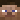Glitchgames Server IP & Reviews | #1 Minecraft Servers ListComing soon is a fun 1.18 survival Minecraft server. Parkour survival hide and seek, events, fun new thing :):):):):):):):):):):):):):):):):):):):):):):):):):):):):):):):):):):):):):):):):):):):):):):):):):):):):):):):) :):):):):):):):):):):):):):):):):):):):):):):):):):):):) :):):):):):):):):):):):):):):):):):):):):):):):):):):):) :):):):):):):):):):):):):):):):):):):):):):):):):):):):) :):):):):):):):):):):):):):):):):):):):):):):):):):):):) :):):):):):):):):):):):):):):):):):):):):):):):):):):):) :):):):):):):):):):):):):):):):):):):):):):):):):):):):) :):):):):):):):):):):):):):):):):):):):):):):):):):):):) :):):):):):):):):):):):):):):):):):):):):):):):):):):):) :):):):):):):):):):):):):):):):):):):):):):):):):):):):) :):):):):):):):):):):):):):):):):):):):):):):):):):):):) :):):):):):):):):):):):):):):):):):):):):):):):):):):):) :):):):):):):):):):):):):):):):):):):):):):):):):):):):) :):):):):):):):):):):):):):):):):):):):):):):):):):):):)

## What is the server IP for Glitchgames?

The IP address for Glitchgames is Glitchgamesmc.shock.gg. You can use this IP Address to start playing on the Glitchgames Minecraft Server now. This is the most recent, accurate, and working IP Address you will find as of 2022.

## How do I play on the Glitchgames Minecraft server?

Open the Minecraft launcher, next click the "Play" button, then select "Multiplayer" from the main menu.

Click the "Add Server" button to open the server information form then input the the IP Address Glitchgamesmc.shock.gg in the "Server Address" box then press click on "Done" button.

Once the connection to the server is verified by Mojang session servers and is available, the connection icon will turn green and you can click on the "Join Server" button to play on Glitchgames and experience their latest updates and gamemodes!

## What Minecraft game version does Glitchgames server support?

Glitchgames supports Minecraft game version: 1.18, however keep in mind that many Minecraft servers allow players to use older or newer versions so please make sure to check Glitchgames Discord or Website for more information on version compatibility.

## Where is the Glitchgames Minecraft Server being hosted?

The Glitchgames server is currently hosted in United States and has a great connection as of the 24 of Mar at 10:25 EST, 2022.

## What gamemodes can I play on the Glitchgames Minecraft Server?

You can play Parkour, Mini Games, Survival, on the Glitchgames Minecraft Server. This gamemode list was updated by the server owner on the 24 of Mar at 10:25 EST, 2022

## What is the Discord server for the Glitchgames Minecraft Server?

You can join the Discord server for Glitchgames by clicking on the following invite link: You can chat with other players, request support, and read about the latest updates in Glitchgames's Discord Server.

## What is the website for the Glitchgames Minecraft Server?

The website link for the Glitchgames server is . On the website, you will find the forums, store, and news for the server.

### Joins by Slug

Sorted by conversion rate. All-time data.

Temporarily disabled.

 Rank Tag Clicks Joins Conversion

### JOINS BY COUNTRY

Sorted number of joins. All-time data.

 Rank Country Joins Percent

YOU CAN VOTE ONCE A DAY!

Viewing the latest 0 reviews
Disclaimer:
• We only count unique IP copies. Meaning only 1 copy from a device/ip combination is counted per day.
• Manual IP copies are not counted. Only clicks on the ip address button are counted.
• Player count data tracking started June 14, 12AM 2022.
• ### All Time

 Rank Username Votes #1itsnoobtastic 1